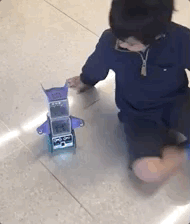## Robotics Camp A (Grades TK-K)The Robotics Camp for Accelerated Math Learning for students in transitional kindergarten and kindergarten enables accelerated and deeper math learning through robotics projects and solving real-world problems using math concepts. The campers will also learn English and math literacy, teamwork, and communication skills.

The curriculum for this camp includes:

• Mathematics​

• Count and Model Numbers​

• Compare Numbers

• Number Line

• Addition and Subtraction

• Measurement

• Represent and Sort Data

• Geometry

• Coding

• Principles of Engineering

• Science

• Art

• Music